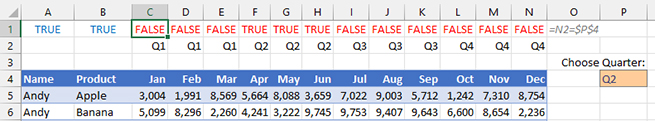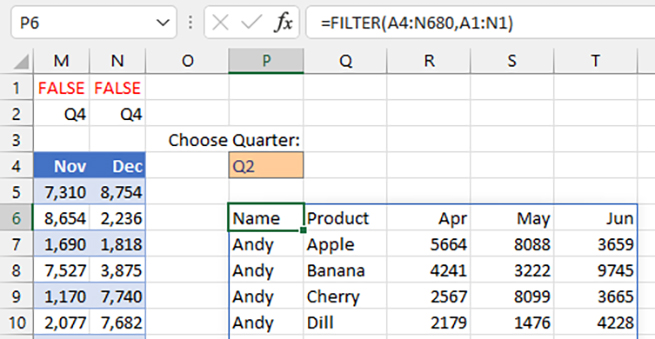In Excel 2019, Microsoft added the FILTER function that allows you to filter using a formula. Unlike the legacy filter commands, the FILTER function offers a barely documented feature—the ability to select certain columns from a data set.

When Microsoft introduced the FILTER function, its demos and examples all focused on retrieving certain rows from a data set. For example, the data in Figure 1 has headings in A4:N4 and data in A5:N680. Microsoft’s example for using FILTER would be to retrieve all rows where the product in column B was equal to “Apple” with the formula =FILTER(A5:N680,B5:B680="Apple").

In this example, A5:N680 is a 676-row and 14-column array of data. The “Include” argument (B5:B680) is a 676-row by 1-column series of True or False values. Any time that column B is equal to “Apple,” that row is returned by the FILTER function.

### FILTER WORKS SIDEWAYS

I’m sometimes asked if the Excel Filter or Advanced Filter commands can work sideways. The answer was always no. But now, using the FILTER function in a formula, you can choose which columns to extract from a data set.

I learned this from Excel MVP Leila Gharani’s amazing video published on her YouTube channel several months after the FILTER function was released. She had discovered that if the Include argument was a 1-row by 14-column array of True and False values, then Excel would apply the filter in a sideways fashion, returning all of the rows and only the columns where the Include value is True.

Let’s say that you wanted a way to extract sales for months that fell in a single, specified quarter. In addition to sales for those months, you would want to retrieve the name and product from the left side of the range.

The first step is to use some formulas to decide which columns to include. In this example, the person using the spreadsheet will be able to type a quarter in cell P4. Data in row 2 identifies the quarter number for each month. Helper formulas in C1:N1 will return the value of TRUE if the month is in the selected quarter by using the formula =C1=\$P\$4.

No matter which quarter is selected, you always want to return the Name and Product columns, so simply enter the value of TRUE in A1 and B1, as shown in Figure 1.The formula that will return the Name, Product, and three months from the selected quarter is =FILTER(A4:N680,A1:N1). The results are shown in Figure 2. Note that it’s okay to include the product headings in the first argument of the function, so you can point to A4:N680. For the Include argument, specify the row of TRUE/FALSE values in A1:N14.

### CAVEAT WITH SPILLABLE FUNCTIONS

The single formula in P6 of Figure 2 is known as a Dynamic Array. One formula produces all of the answers shown in P6:T13. This is powerful, but there’s one problem: Excel isn’t smart enough to copy the formatting from the original cells to the spilled array. In the current example, that means that you’re losing the thousands separator (“5,664”) from the original data. The value shows up in the spilled array as “5664” instead. This may not seem like a big issue in this case. But if the original data had included dates such as 01/23/2023, the spilled values would lose their date formatting and the underlying serial number of 44927 would show up instead.The workaround is to plan on reapplying the formatting to the spilled array. In this case, applying a number format of #,##0 to R6:U682 would restore the thousands separators.